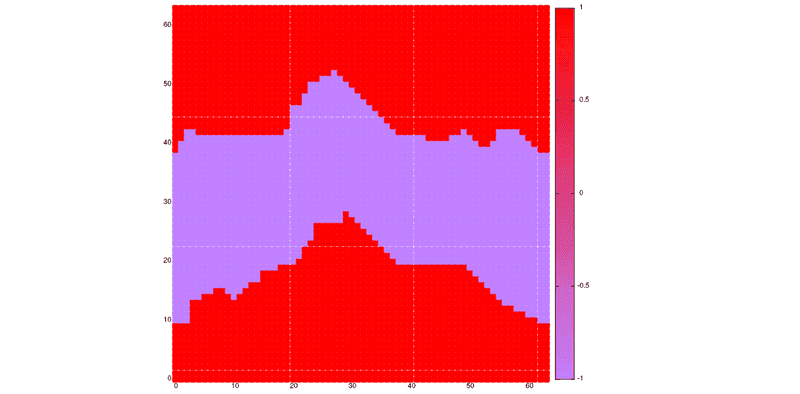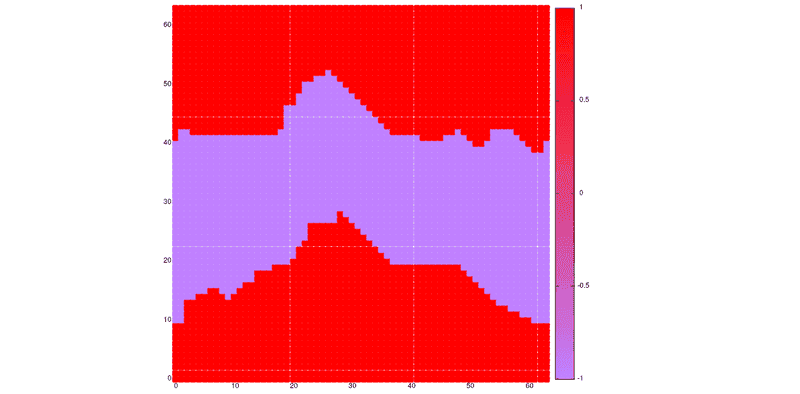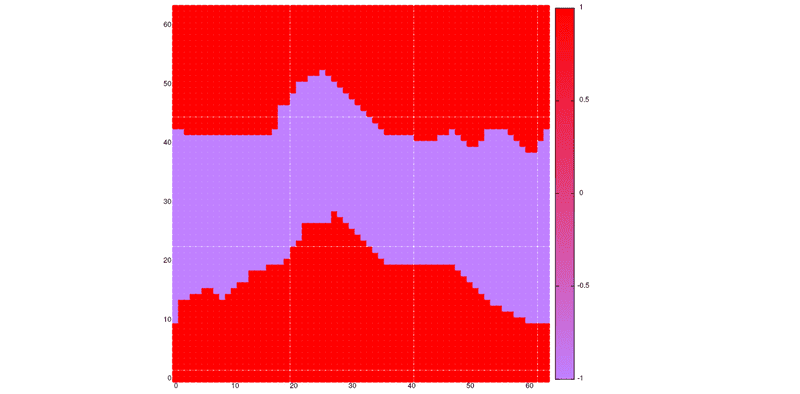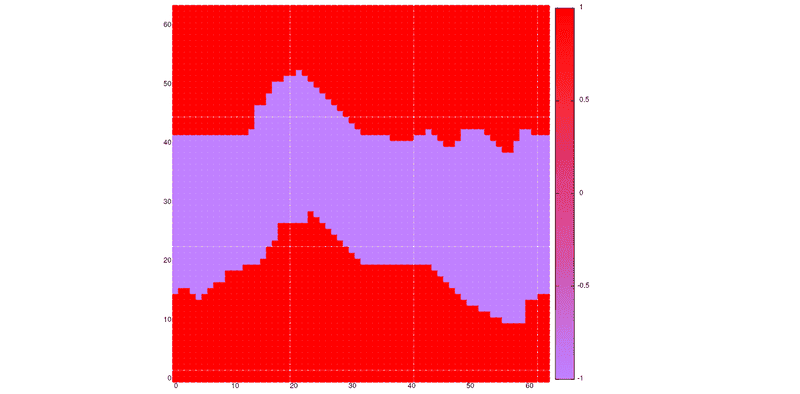# Weird fluctuations in Ising model

• C/++/#
Not 100% sure this thread belongs in this section, sorry if it's out of place.

I was trying to run a simulation of a 2D Ising model; i thought everything was going fine until I started to look at the numerical results and I noticed that I get some wild fluctuations in my results; as an example, here are the values i get for final energy and magnetization (per site) after 10 different 10000-step runs on a 128x128 lattice at temperature T = 0.1 K(critical temperature is at 2.27 K), where the initial spin configuration is chosen randomly(+1 or -1 is assigned to each node with equal probability):
$$(E, |M|) = (-2, 1); (-1.95, 0.52); (-2, 1); (-1.95, 0.21); (-2, 1); (-1.95, 0.02); (-2, 1); (-1.95; 0.24); (-2, 1); (-2; 1)$$
With a temperature so low I would expect all $|M|$ to be exactly 1, or at least that fluctuations like these would be way less probable than 4/10, so I guess there must be some error in my code. Am I wrong in thinking this?
I would be very grateful if someone could take a look at my code and maybe point out where the error is, cause it seems like I can't find it.

Here is the function used to update the lattice(it's Metropolis algorithm):
Code:
void update(graph *pLattice, int *s, double *prob, int *pE, int *pM){
/* pLattice is the pointer to the graph/lattice; s is the pointer
* to the array containing the spin value for each site; prob is the pointer to
* the lookup table used to map differences in energy to their probability according
* to Boltzmann distribution; pE and pM point respectively to the energy and
* magnetization values of the lattice. */
int k, site, *pNeigh, siteConn, sum;
for(site = 0; site < pLattice->numNodes; site++){ // loop over all nodes
pNeigh = pLattice->neigh[site]; // = neigh[site]
siteConn = pLattice->conn[site]; // = 4
sum = 0;
for(k = 0; k < siteConn; k++, pNeigh++){
sum += s[*pNeigh];  // = s[neigh[site][k]]
}
sum *= s[site]; // this is now the energy of site
if(sum <= 0 || drand48() < prob[sum]){
/* this is legit because sum = half the variation of energy due to the flip of s[site]
* and 0 < prob[sum] = exp(-2.0 * sum / T) < 1; (T=temperature) */
s[site] = -s[site];
*pE += 2*sum;
*pM += 2*s[site];
}
}
return;
}
"graph" is basically an adjacency list, defined as
Code:
typedef struct {
/* conn points to an array of size numNodes, whose items are the number of
* connections of each node(i'm using periodic bc so conn[i] = 4 for every value of i).
* neigh points to a 2d array of dimensions numNodes*numLinks,
* where the neighbours of each node are stored. */
} graph;
The main function, besides declaration of variables and error checking, is basically just
Code:
  E = energy(pLattice, s);
M = magnetization(pLattice, s);
for(i=0;i<R;i++){
update(pLattice, s, probLUT, &E, &M);
}
fprintf(stderr, "E = %f; M = %f\n", (double)E/N, (double)M/N);
where N is the number of nodes.

The difference in energy value between "correct" and "weird" runs is small, so when I average over lot of runs for each T, and for many values of T, the plot EvsT
looks not too different from the expected curve; the plot of |M|vsT still shows the correct behaviour, but instead of getting constant |M|=1 for $T<T_c$ i get values oscillating around |M|=0.8. I tried running for different times, different lattice size, different initial conditions, with no different results.
I'll post full code, graphs or whatever if needed. Thanks.

Updating just in case anyone happens to come by and help me out. I created some animated plots for the 'weird' results and I found that they correspond to situations where two connected clusters(one for each spin) arise and reach some sort of equilibrium configuration where they are just translated (always) in the $-x$ direction through the periodic boundary. Here a few pics of a 64x64 lattice taken at times 850,851,852,856: